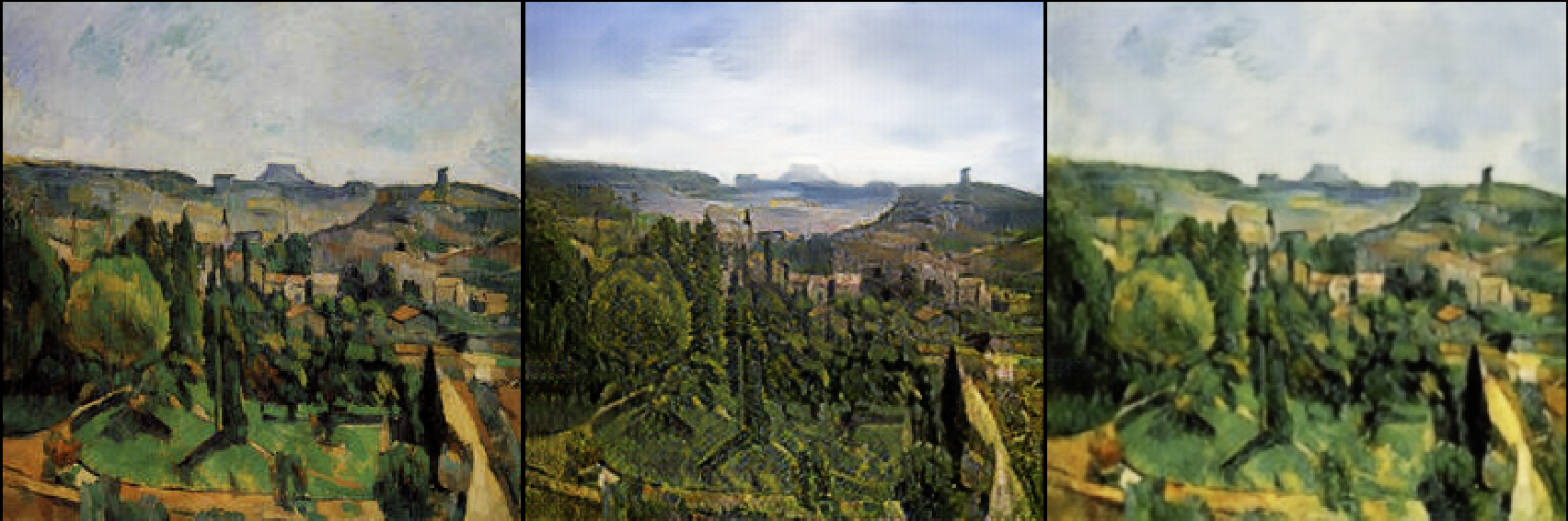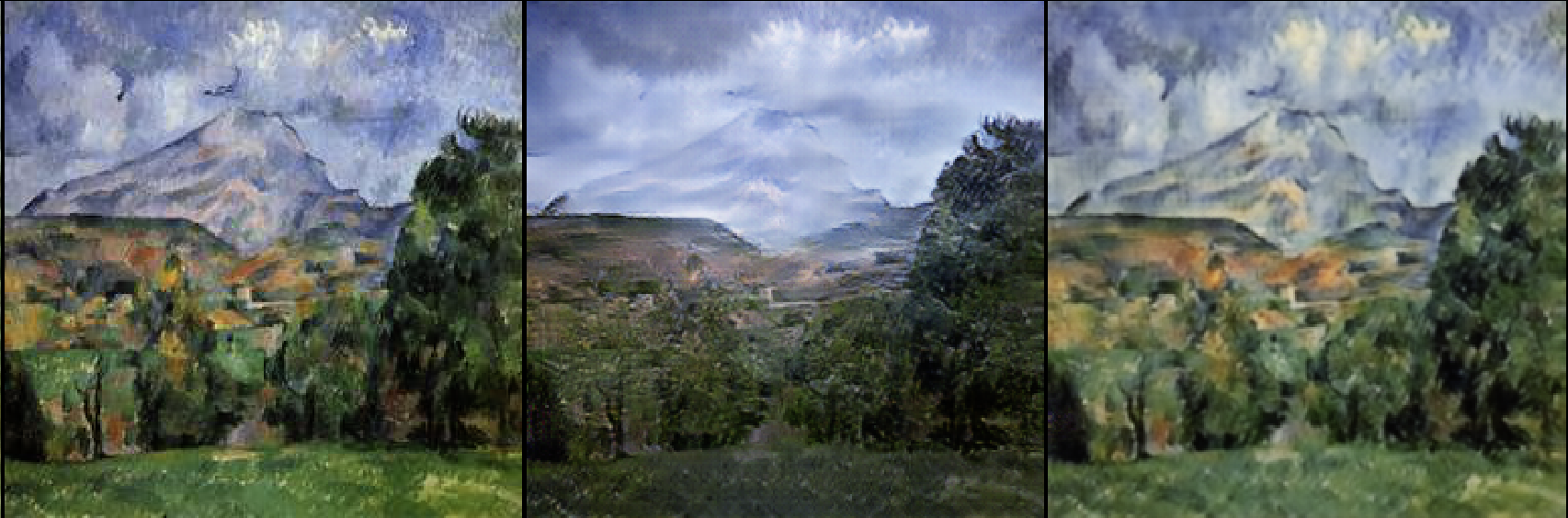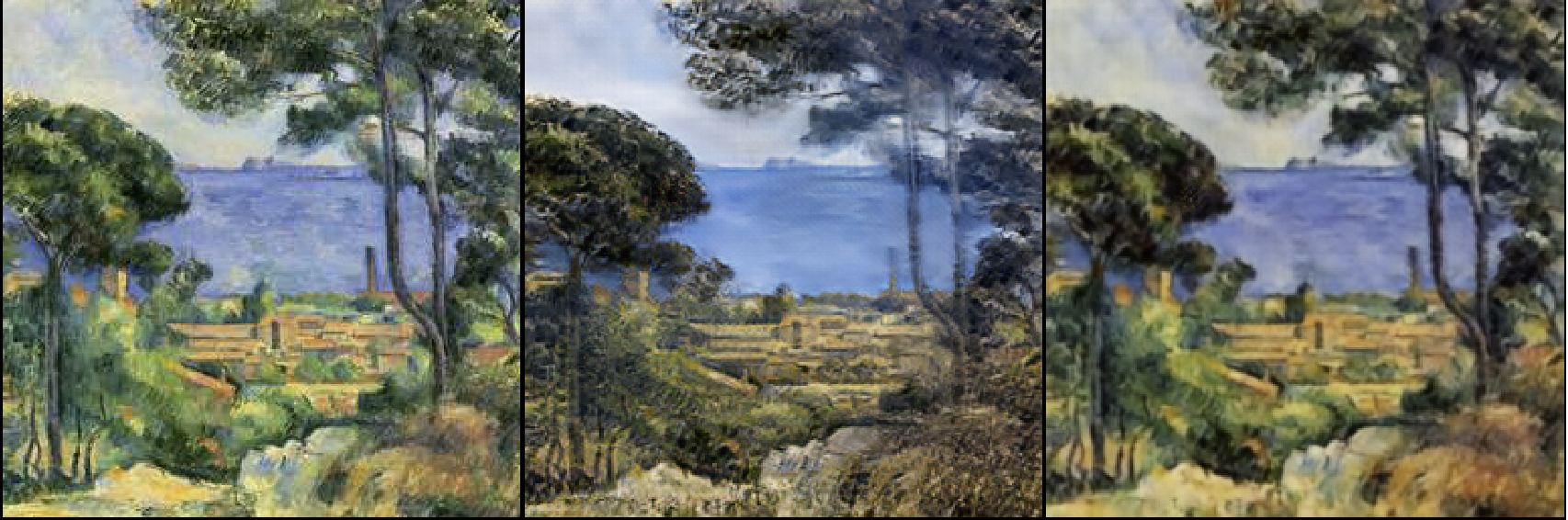Need help with AI-Art?
Click the “chat” button below for chat support from the developer who created it, or find similar developers for support.Adi-iitd227 Stars43 ForksMIT License308 Commits2 Opened issues#### Description

PyTorch (and PyTorch Lightning) implementation of Neural Style Transfer, Pix2Pix, CycleGAN, and Deep Dream!!
?

#### Need anything else?No Data

# AI Art

### Edit 2020/11/20:

Support of PyTorch Lightning added to Neural Style Transfer, CycleGAN and Pix2Pix. Thanks to @William!

Why PyTorch Lightning? - Easy to reproduce results - Mixed Precision (16 bit and 32 bit) training support - More readable by decoupling the research code from the engineering - Less error prone by automating most of the training loop and tricky engineering - Scalable to any hardware without changing the model (CPU, Single/Multi GPU, TPU)

## Motivation

Creativity is something we closely associate with what it means to be human. But with digital technology now enabling machines to recognize, learn from, and respond to humans, an inevitable question follows: Can machines be creative?

It could be argued that the ability of machines to learn what things look like, and then make convincing new examples marks the advent of creative AI. This tutorial will cover four different Deep Learning models to create novel arts, solely by code - Style Transfer, Pix2Pix, CycleGAN.

## Neural Style Transfer

Style Transfer is one of the most fun techniques in Deep learning. It combines the two images, namely, a Content image (C) and a Style image (S), to create an Output image (G). The Output image has the content of image C painted in the style of image S.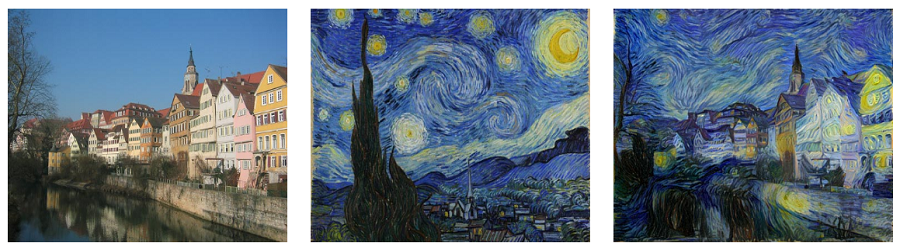Style Transfer uses a pre-trained Convolutional Neural Network to get the content and style representations of the image, but why do these intermediate outputs within the pre-trained image classification network allow us to define style and content representations?

These pre-trained models trained on image classification tasks can understand the image very well. This requires taking the raw image as input pixels and building an internal representation that converts the raw image pixels into a complex understanding of the features present within the image. The activation maps of first few layers represent low-level features like edges and textures; as we go deeper and deeper through the network, the activation maps represent higher-level features - objects like wheels, or eyes, or faces. Style Transfer incorporates three different kinds of losses:

• Content Cost: JContent (C, G)
• Style Cost: JStyle (S, G)
• Total Variation (TV) Cost: JTV (G)

Putting all together: JTotal (G) = α x JContent (C, G) + β x JStyle (S, G) + γ x JTV (G). Let's delve deeper to know more profoundly what's going on under the hood!

### Content Cost

Usually, each layer in the network defines a non-linear filter bank whose complexity increases with the position of the layer in the network. Content loss tries to make sure that the Output image G has similar content as the Input image C, by minimizing the L2 distance between their activation maps.

Practically, we get the most visually pleasing results if we choose a layer in the middle of the network - neither too shallow nor too deep. The higher layers in the network capture the high-level content in terms of objects and their arrangement in the input image but do not constrain the exact pixel values of the reconstruction very much. In contrast, reconstructions from the lower layers simply reproduce the exact pixel values of the original image.

Let a(C) be the hidden layer activations which is a Nh x Nw x Nc dimensional tensor, and let a(G) be the corresponding hidden layer activations of the Output image. Finally, the Content Cost function is defined as follows: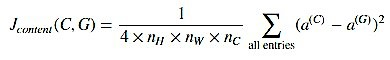Nh, Nw, Nc are the height, width, and the number of channels of the hidden layer chosen. To compute the cost JContent (C, G), it might also be convenient to unroll these 3D volumes into a 2D matrix, as shown below.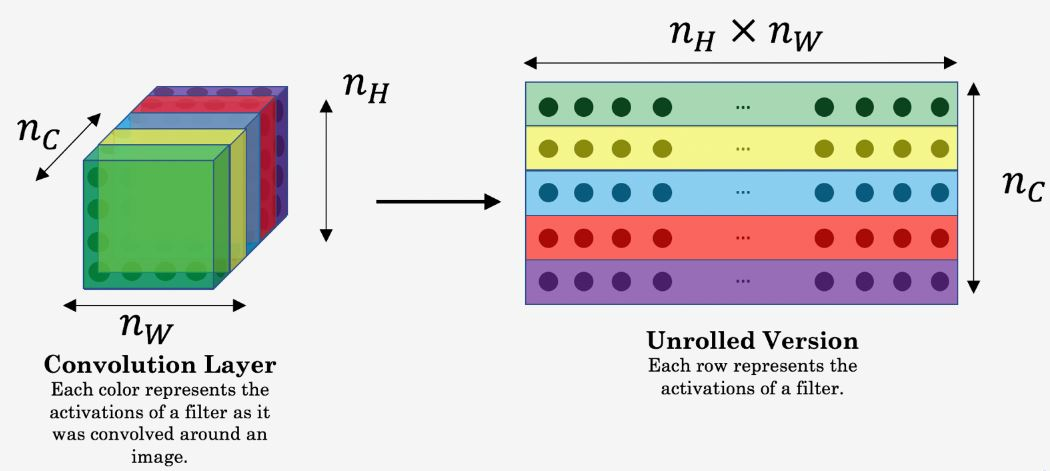The first image is the original one, while the remaining ones are the reconstructions when layers Conv_1_2, Conv_2_2, Conv_3_2, Conv_4_2, and Conv_5_2 (left to right and top to bottom) are chosen in the Content loss.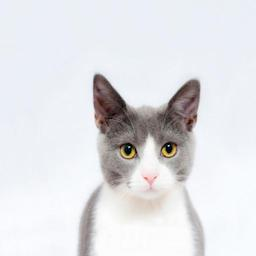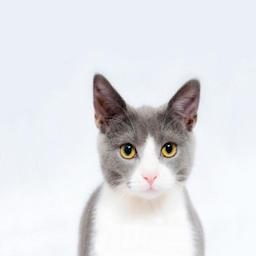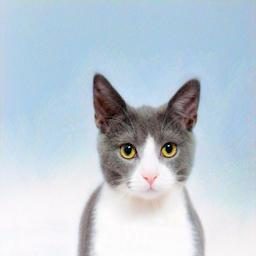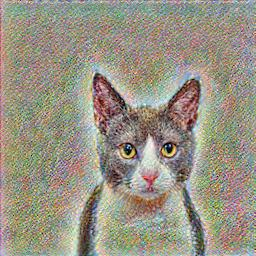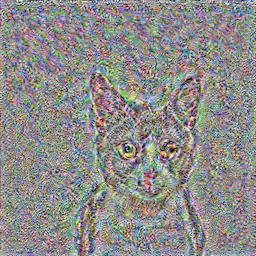### Style Cost

To understand it better, we first need to know something about the Gram Matrix . In linear algebra, the Gram matrix G of a set of vectors (v1, …, vn) is the matrix of dot products, whose entries are G(i, j) = np.dot(vi, vj). In other words, G(i, j) compares how similar vi is to vj. If they are highly similar, the outcome would be a large value, otherwise, it would be low suggesting a lower correlation. In Style Transfer, we can compute the Gram matrix by multiplying the unrolled filter matrix with its transpose as shown below: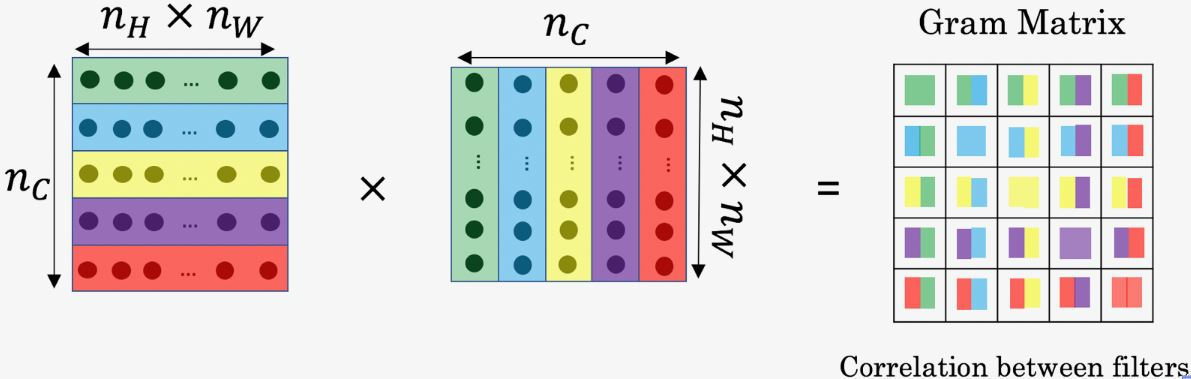The result is a matrix of dimension (nC, nC) where nC is the number of filters. The value G(i, j) measures how similar the activations of filter i are to the activations of filter j. One important part of the gram matrix is that the diagonal elements such as G(i, i) measures how active filter i is. For example, suppose filter i is detecting vertical textures in the image, then G(i, i) measures how common vertical textures are in the image as a whole.

By capturing the prevalence of different types of features G(i, i), as well as how much different features occur together G(i, j), the Gram matrix G measures the Style of an image. Once we have the Gram matrix, we minimize the L2 distance between the Gram matrix of the Style image S and the Output image G. Usually, we take more than one layers in account to calculate the Style cost as opposed to Content cost (which only requires one layer), and the reason for doing so is discussed later on in the post. For a single hidden layer, the corresponding style cost is defined as: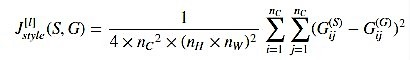### Total Variation (TV) Cost

It acts like a regularizer that encourages spatial smoothness in the generated image (G). This was not used in the original paper proposed by Gatys et al., but it sometimes improves the results. For 2D signal (or image), it is defined as follows: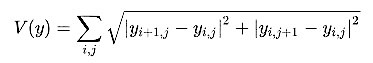### Experiments

> What happens if we zero out the coefficients of the Content and TV loss, and consider only a single layer to compute the Style cost?

As many of you might have guessed, the optimization algorithm will now only minimize the Style cost. So, for a given Style image , we will see the different kinds of brush-strokes (depending on the layer used) that the model will try to enforce in the final generated image (G). Remember, we started with a single layer in the Style cost, so, running the experiments for different layers would give different kinds of brush-strokes. Suppose the style image is famous The great wall of Kanagawa shown below: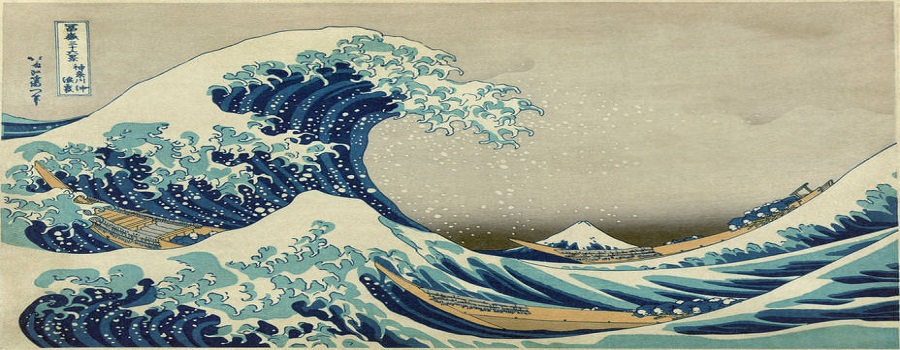The brush-strokes that we get after running the experiment taking different layers one at a time are attached below.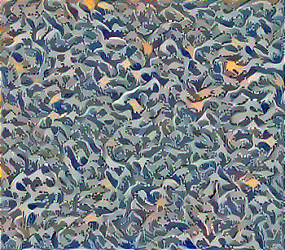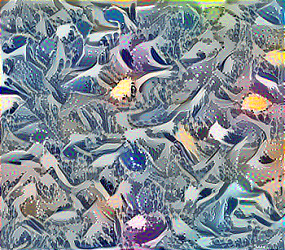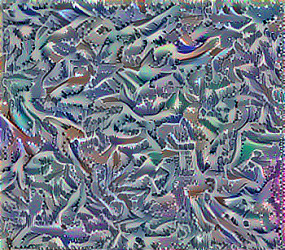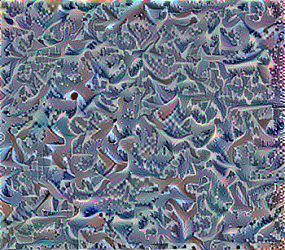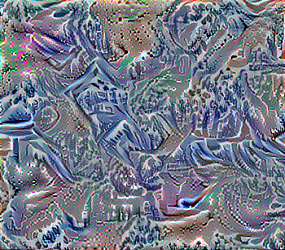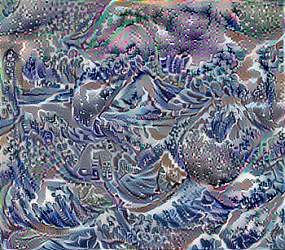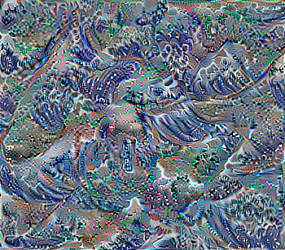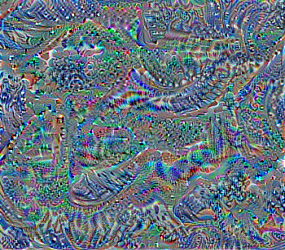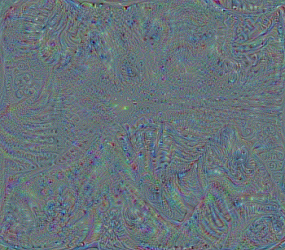These are brush-strokes that the model learned when layers Conv_2_2, Conv_3_1, Conv_3_2, Conv_3_3, Conv_4_1, Conv_4_3, Conv_4_4, Conv_5_1, and Conv_5_4 (left to right and top to bottom) were used one at a time in the Style cost.

The reason behind running this experiment was that the authors of the original paper gave equal weightage to the styles learned by different layers while calculating the Total Style Cost. Now, that's not intuitive at all after looking at these images, because we can see that styles learned by the shallower layers are much more aesthetically pleasing, compared to what deeper layers learned. So, we would like to assign a lower weight to the deeper layers and higher to the shallower ones (exponentially decreasing the weightage could be one way).

### Results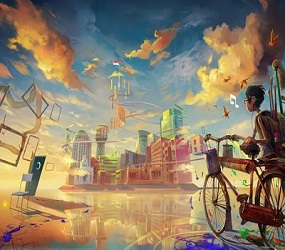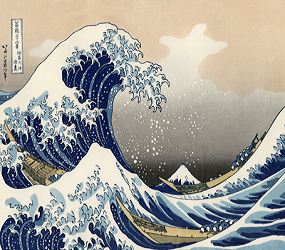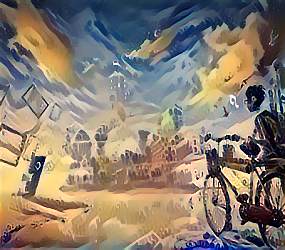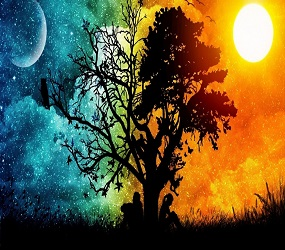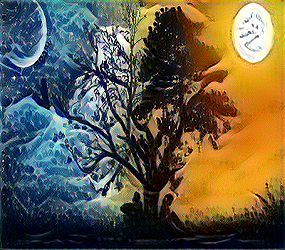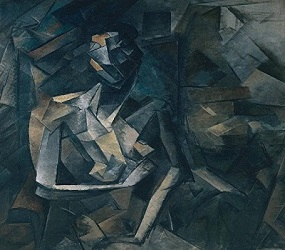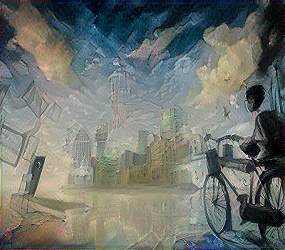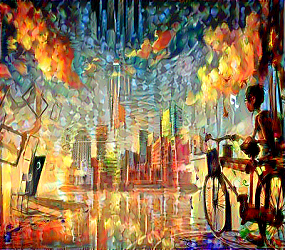## Pix2Pix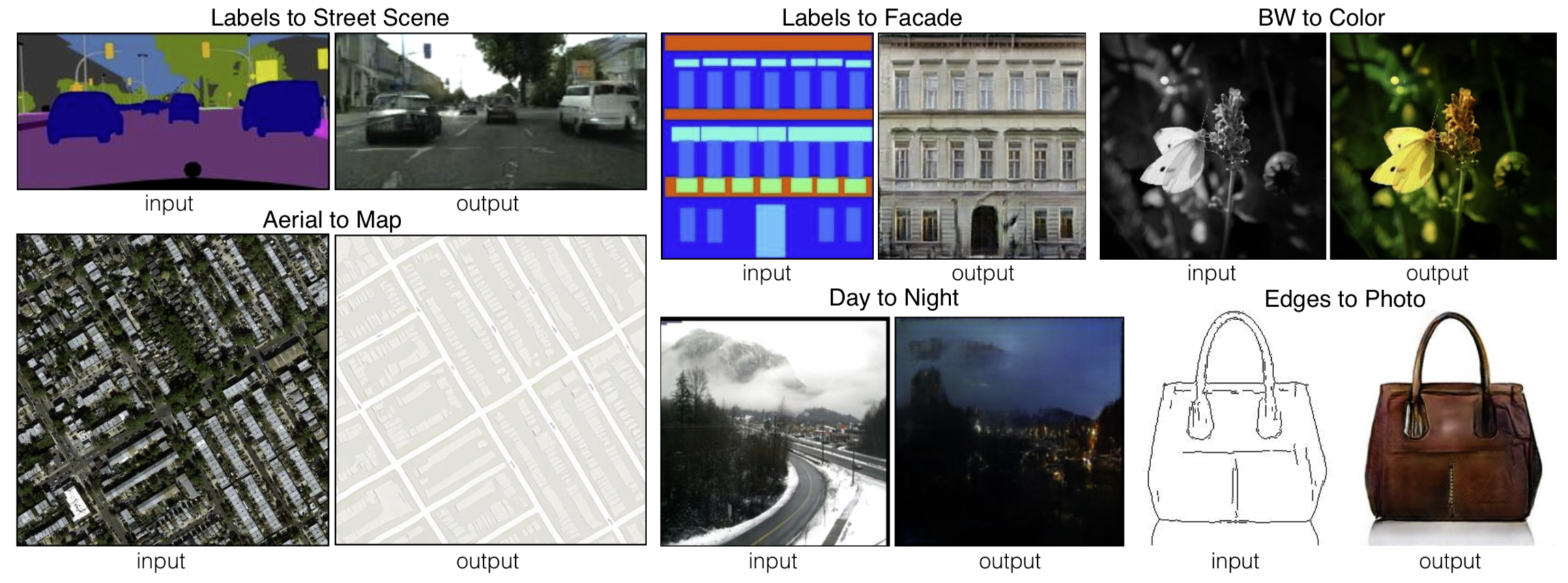> If you don't know what Generative Adversarial networks are, please refer to this blog before going ahead; it explains the intuition and mathematics behind the GANs.

Authors of this paper investigated Conditional adversarial networks as a general-purpose solution to Image-to-Image Translation problems. These networks not only learn the mapping from the input image to output image but also learn a loss function to train this mapping. If we take a naive approach and ask CNN to minimize just the Euclidean distance between predicted and ground truth pixels, it tends to produce blurry results; minimizing Euclidean distance averages all plausible outputs, which causes blurring.

In Generative Adversarial Networks settings, we could specify only a high-level goal, like “make the output indistinguishable from reality”, and then it automatically learns a loss function appropriate for satisfying this goal. The conditional generative adversarial network, or cGAN for short, is a type of GAN that involves the conditional generation of images by a generator model. Like other GANs, Conditional GAN has a discriminator (or critic depending on the loss function we are using) and a generator, and the overall goal is to learn a mapping, where we condition on an input image and generate a corresponding output image. In analogy to automatic language translation, automatic image-to-image translation is defined as the task of translating one possible representation of a scene into another, given sufficient training data.

Most formulations treat the output space as “unstructured” in the sense that each output pixel is considered conditionally independent from all others given the input image. Conditional GANs instead learn a structured loss. Structured losses penalize the joint configuration of the output. Mathematically, CGANs learn a mapping from observed image X and random noise vector z, to y, G: {x,z} → y. The generator G is trained to produce output that cannot be distinguished from the real images by an adversarially trained discriminator, D, which in turn is optimized to perform best at identifying the fake images generated by the generator. The figure shown below illustrates the working of GAN in the Conditional setting.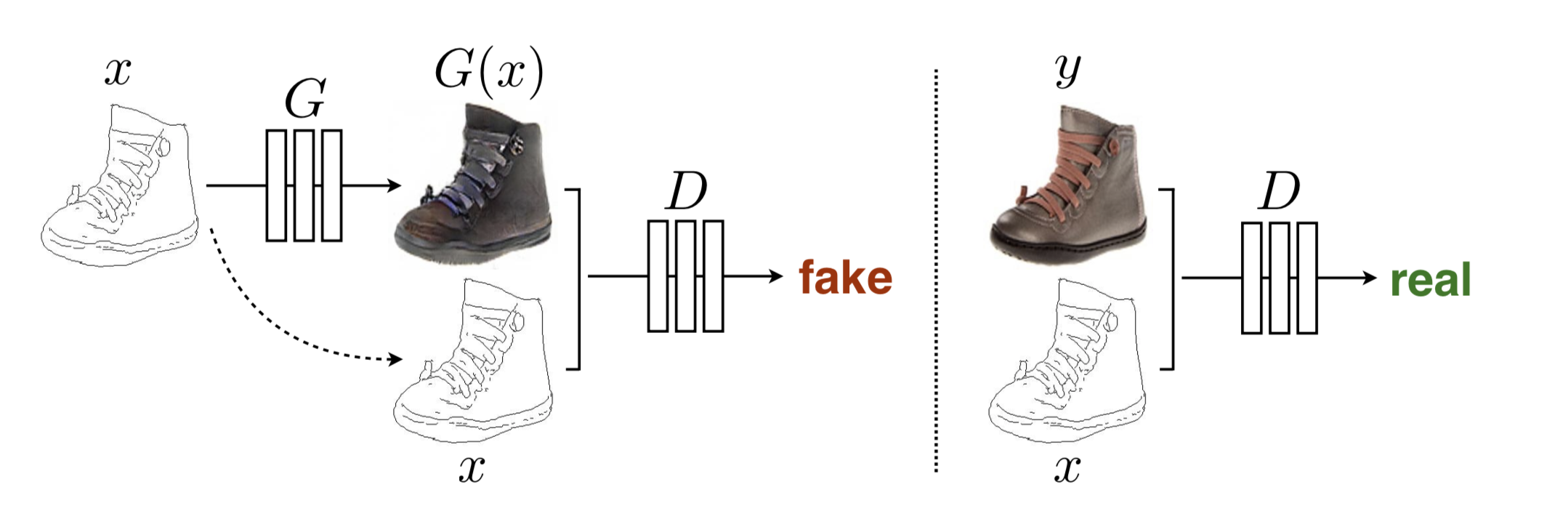### Loss Function

The objective of a conditional GAN can be expressed as:

``` LcGAN (G,D) = Ex,y [log D(x, y)] + Ex,z [log (1 − D(x, G(x, z))], ```

where G tries to minimize this objective against an adversarial D that tries to maximize it. It is beneficial to mix the GAN objective with a more traditional loss, such as L1 distance to make sure that, the ground truth and the output are close to each other in L1 sense. ``` LL1 (G) = Ex,y,z [ ||y − G(x, z)||1 ]. ```

Without z, the net could still learn a mapping from x to y, but would produce deterministic output, and therefore would fail to match any distribution other than a delta function. So, the authors provided noise in the form of dropout; applied it on several layers of the generator at both the training and test time. Despite the dropout noise, there is only minor stochasticity in the output. The complete objective is now, ``` G∗ = arg minG maxD LcGAN (G,D) + λLL1 (G) ```

The Min-Max objective mentioned above was proposed by Ian Goodfellow in 2014 in his original paper, but unfortunately, it doesn't perform well because of vanishing gradients problem. Since then, there has been a lot of development, and many researchers have proposed different kinds of loss formulations (LS-GAN, WGAN, WGAN-GP) to alleviate vanishing gradients. Authors of this paper used Least-square objective function while optimizing the networks, which can be expressed as:

``` min LLSGAN (D) = 1/2 Ex,y [(D(x, y) - 1)2] + 0.5 * Ex,z [D(x, G(x, z))2] ```
``` min LLSGAN (G) = 1/2 Ex,z [(D(x, G(x, z)) - 1)2] ```

### Network Architecture

#### Generator:

Assumption: The input and output differ only in surface appearance and are renderings of the same underlying structure. Therefore, structure in the input is roughly aligned with the structure in the output. The generator architecture is designed around these considerations only. For many image translation problems, there is a great deal of low-level information shared between the input and output, and it would be desirable to shuttle this information directly across the net. To give the generator a means to circumvent the bottleneck for information like this, skip connections are added following the general shape of a U-Net.

Specifically, skip connections are added between each layer i and layer n − i, where n is the total number of layers. Each skip connection simply concatenates all channels at layer i with those at layer n − i. The U-Net encoder-decoder architecture consists of Encoder: ` C64-C128-C256-C512-C512-C512-C512-C512`, and U-Net Decoder: ` C1024-CD1024-CD1024-CD1024-C512-C256-C128,` where Ck denote a Convolution-BatchNorm-ReLU layer with k filters, and CDk denotes a Convolution-BatchNorm-Dropout-ReLU layer with a dropout rate of 50%.

#### Discriminator:

The GAN discriminator models high-frequency structure term, and relies on the L1 term to force low-frequency correctness. To model high-frequencies, it is sufficient to restrict the attention to the structure in local image patches. Therefore, discriminator architecture was termed PatchGAN – that only penalizes structure at the scale of patches. This discriminator tries to classify if each N × N patch in an image is real or fake. The discriminator is run convolutionally across the image, and the responses get averaged out to provide the ultimate output.

Patch GANs discriminator effectively models the image as a Markov random field, assuming independence between pixels separated by more than a patch diameter. The receptive field of the discriminator used was 70 x 70 and was performing best compared to other smaller and larger receptive fields. ` The 70 x 70 discriminator architecture is: C64 - C128 - C256 - C512 `

The diagrams attached below show the forward and backward propagation through the generator and discriminator!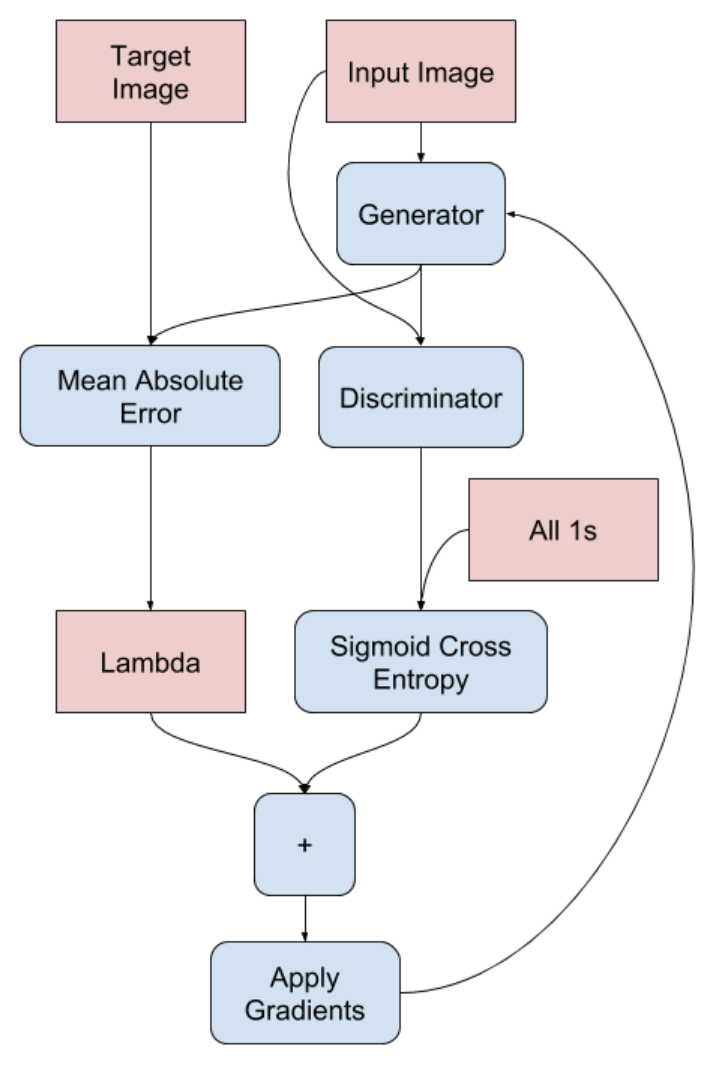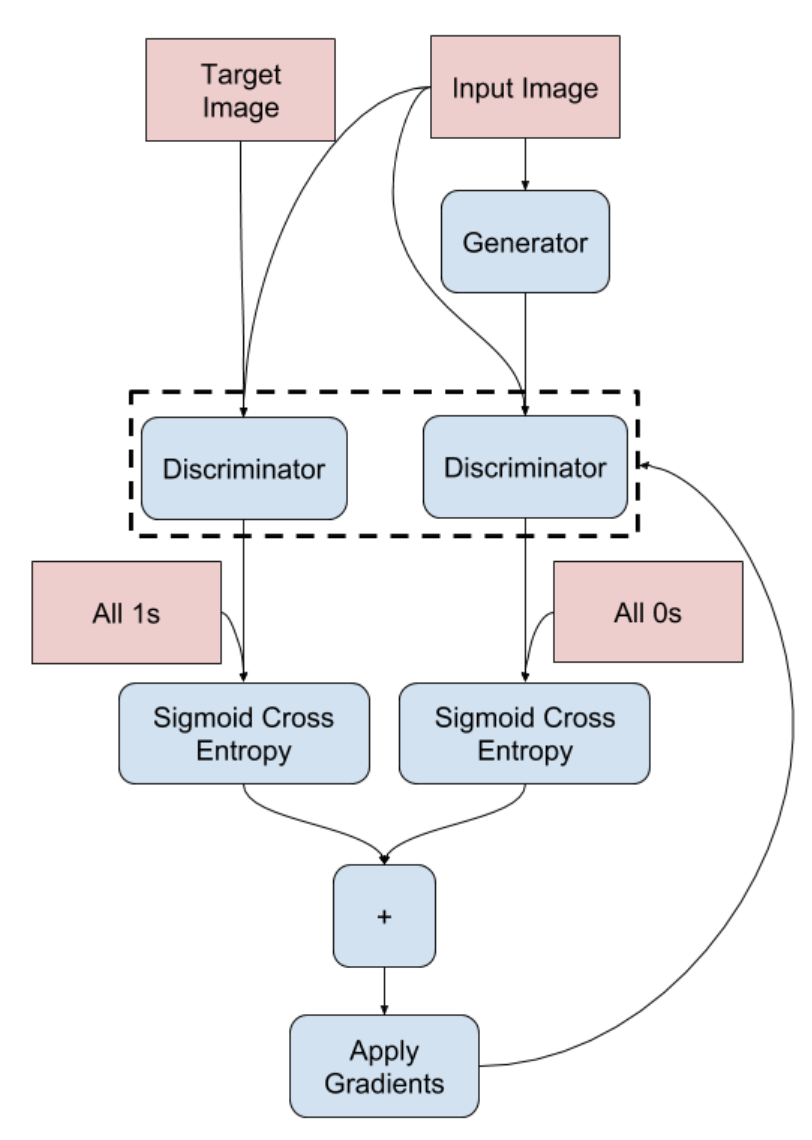### Training Details:

• All convolution kernels are of size 4 × 4.
• Dropout is used both at the training and test time.
• Instance normalization is used instead of batch normalization.
• Normalization is not applied to the first layer in the encoder and discriminator.
• Adam is used with a learning rate of 2e-4, with momentum parameters β1 = 0.5, β2 = 0.999.
• All ReLUs in the encoder and discriminator are leaky, with slope 0.2, while ReLUs in the decoder are not leaky.

### Results

#### Cityscapes: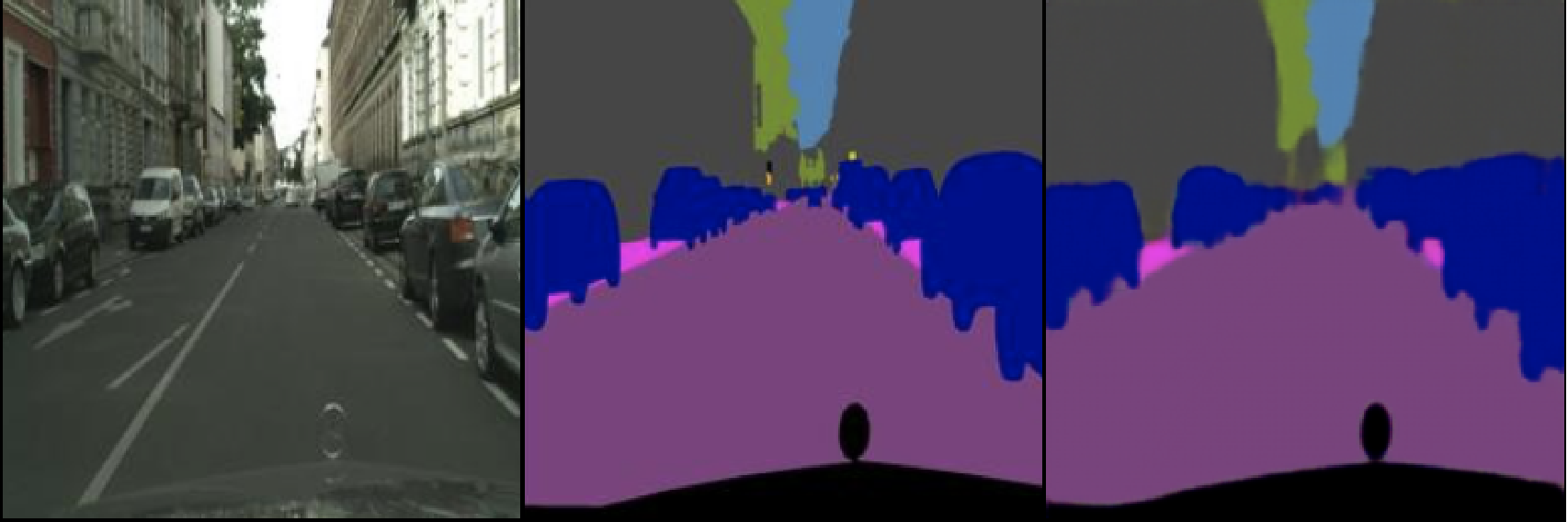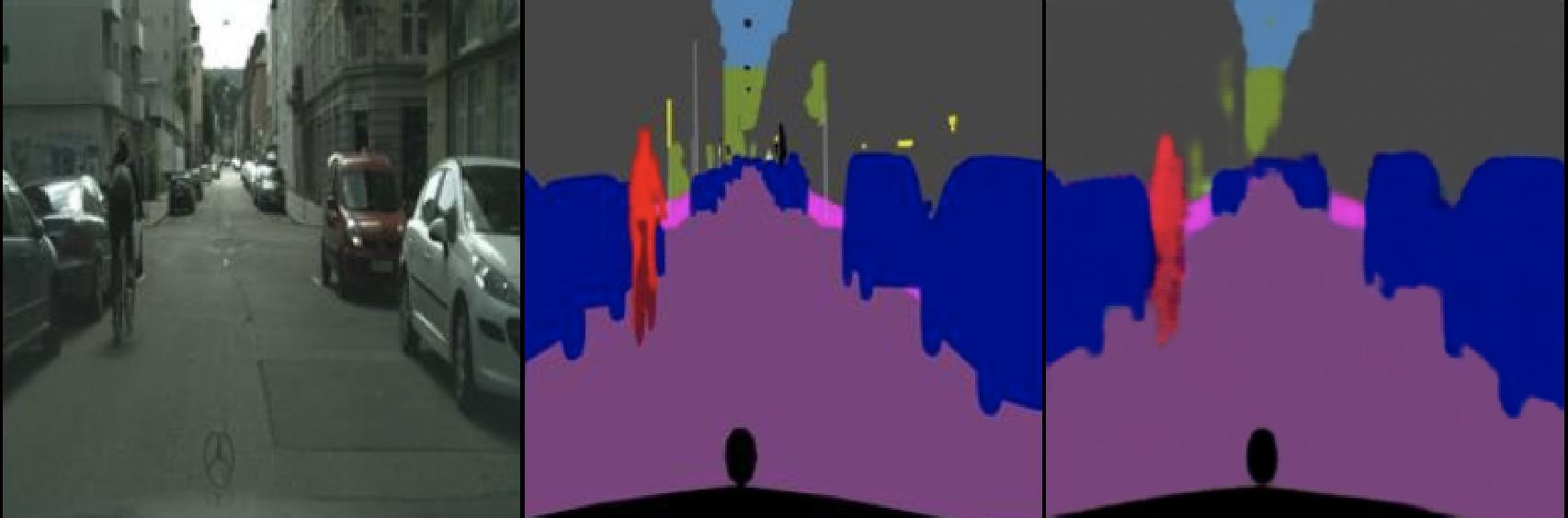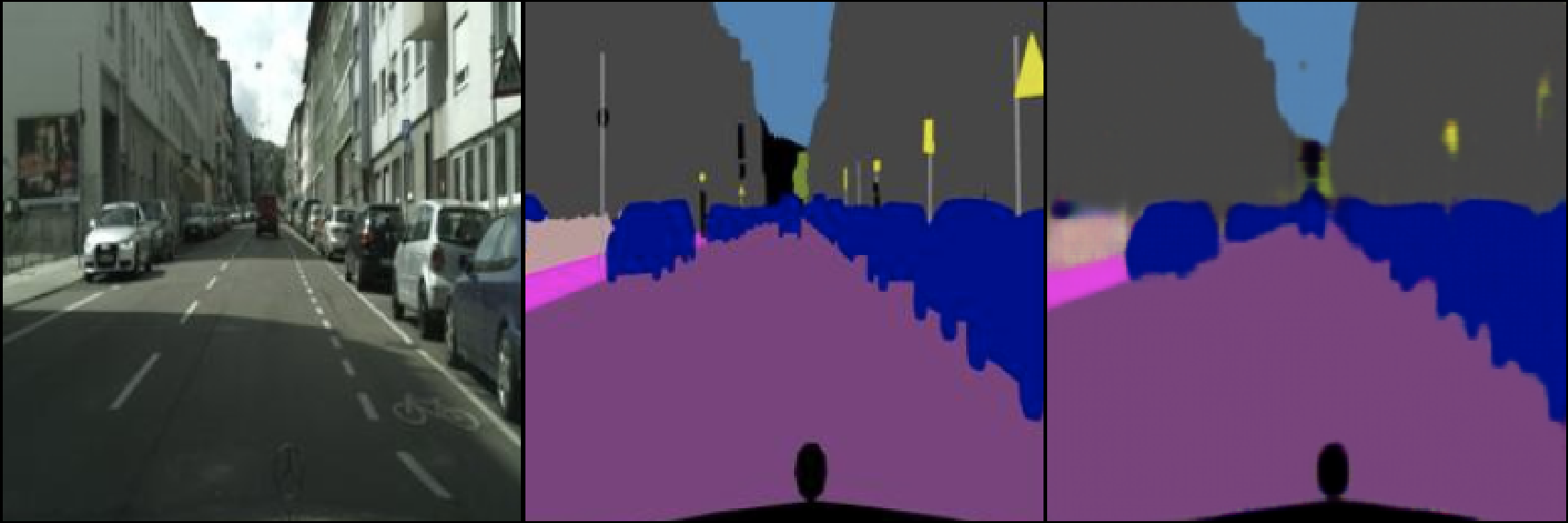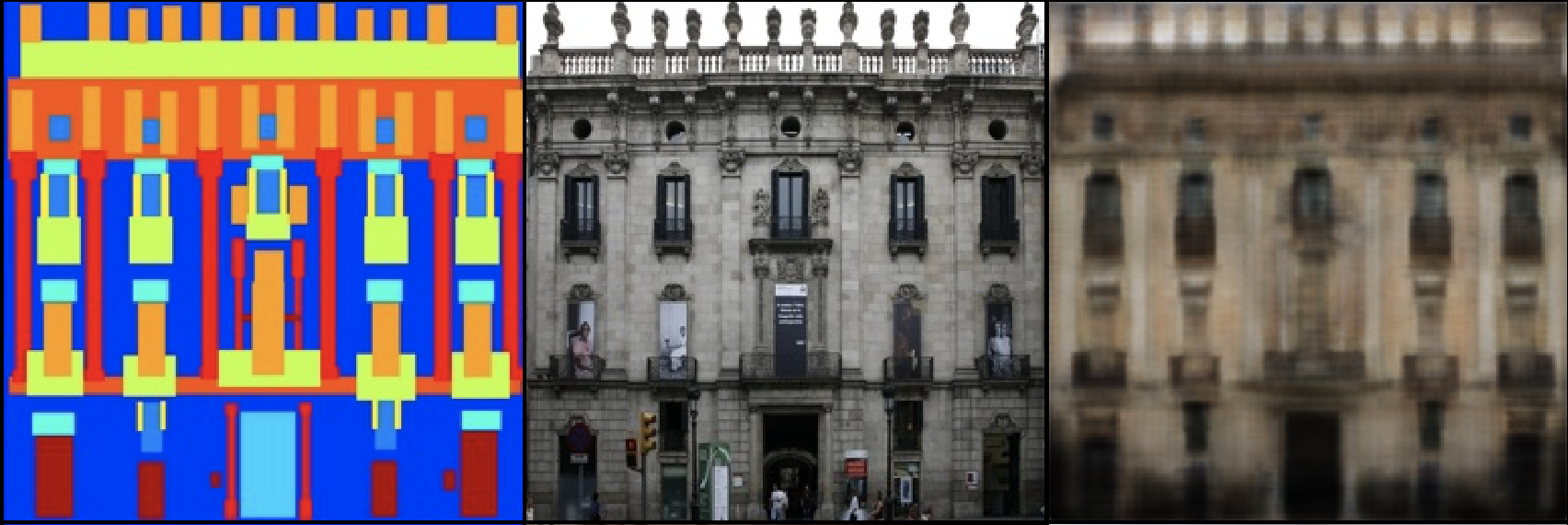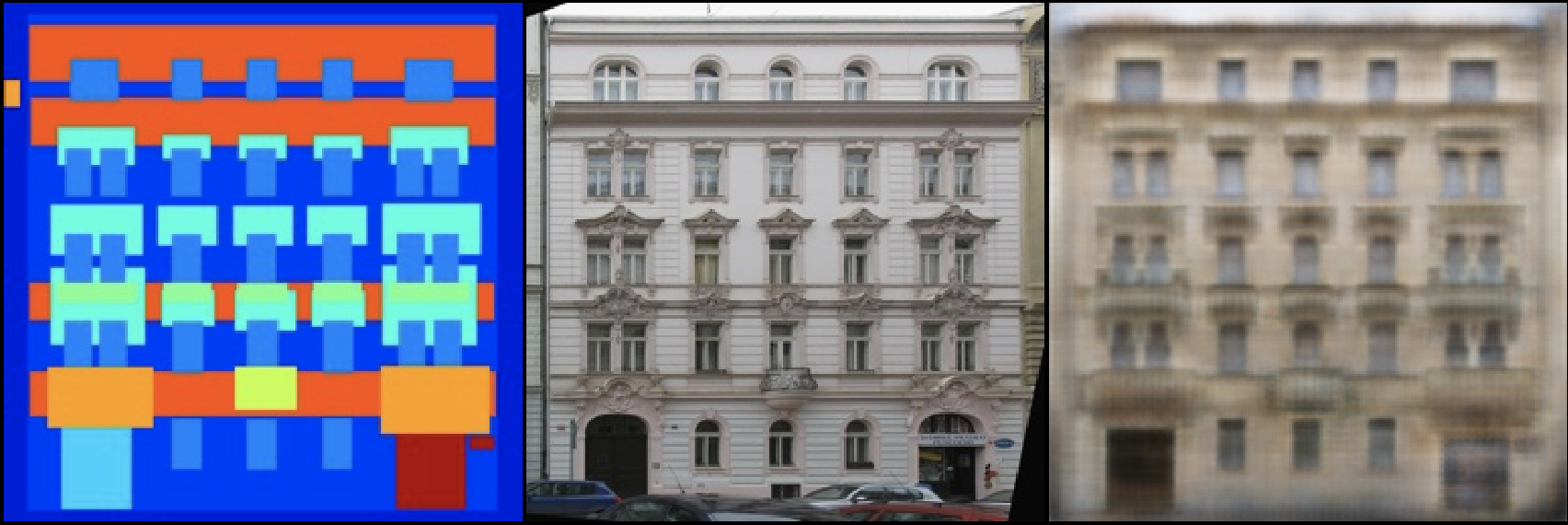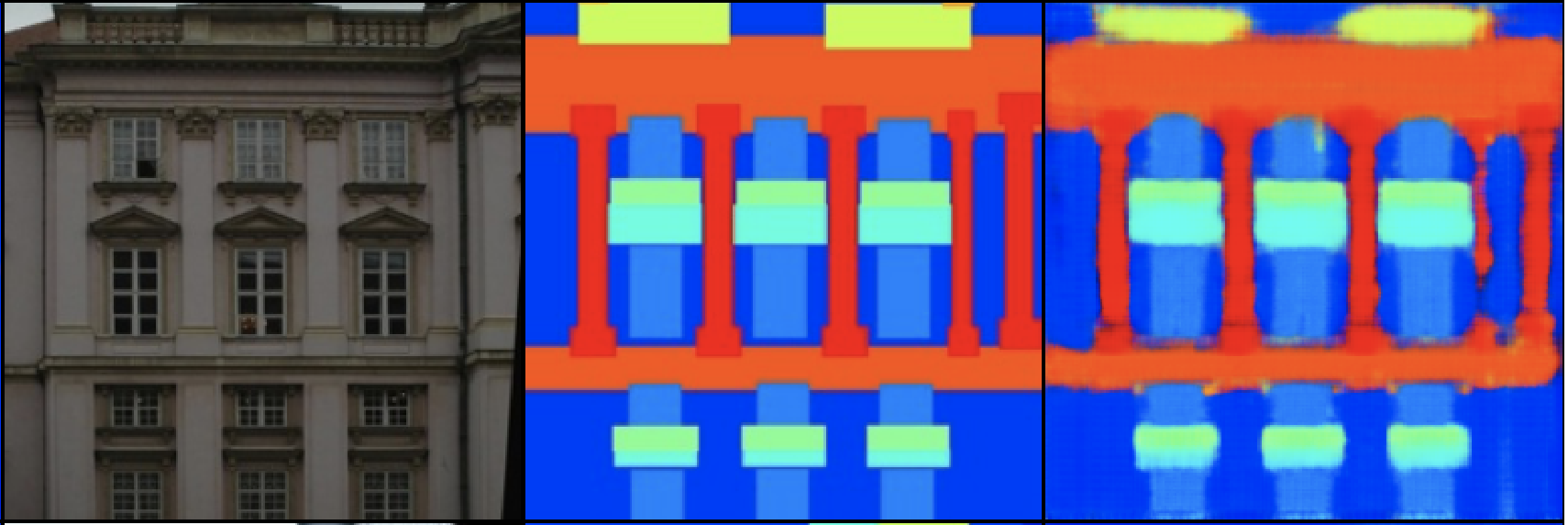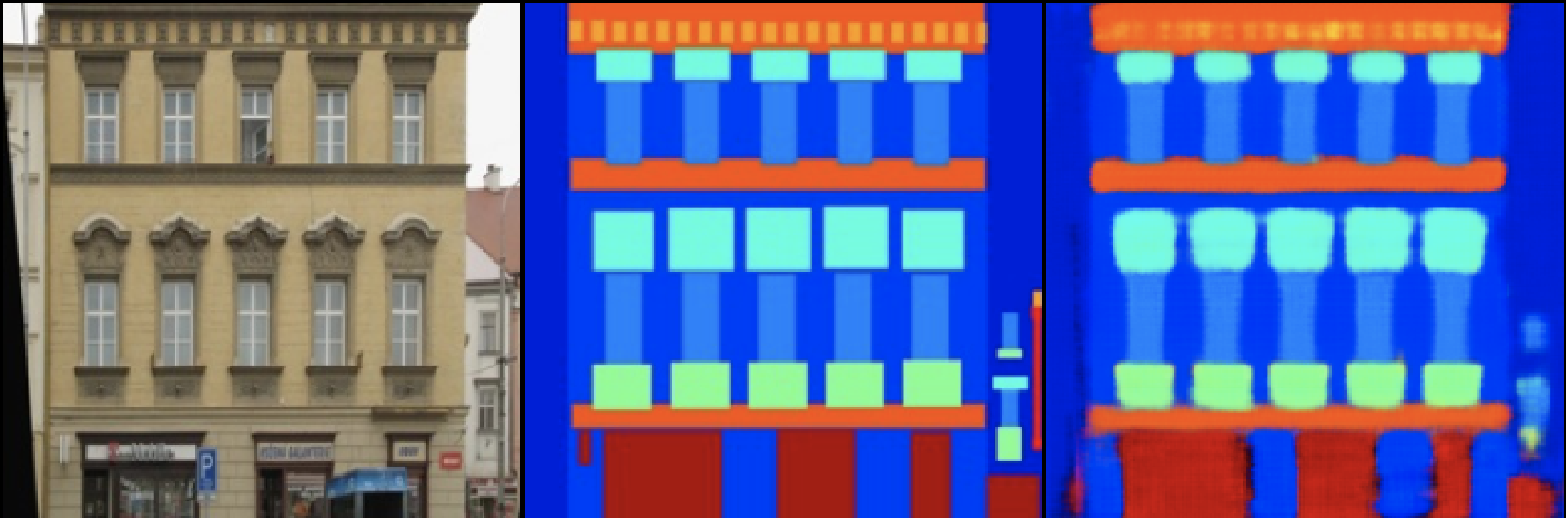## CycleGAN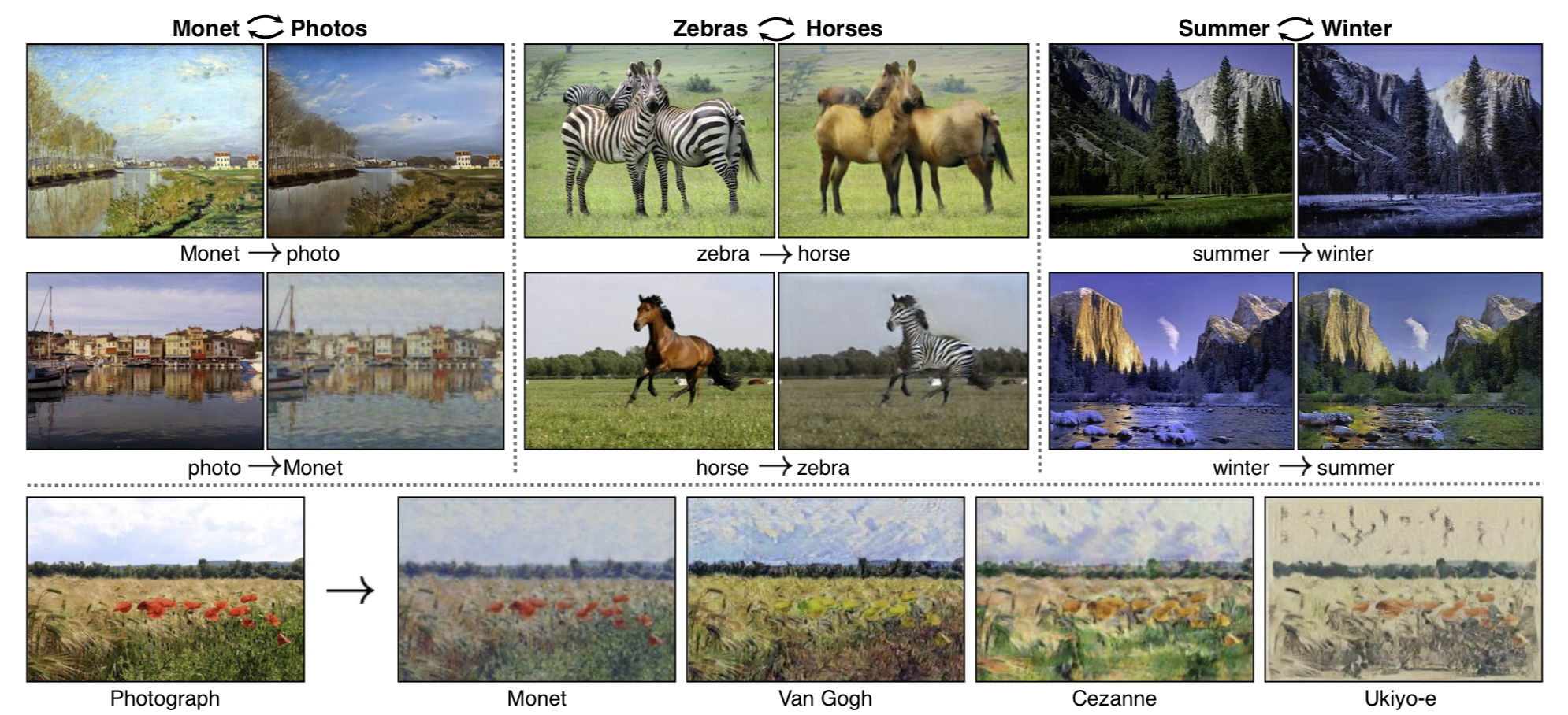The image-to-Image translation is a class of vision and graphics problems where the goal is to learn the mapping between an input image and an output image using a training set of aligned image pairs. However, for many tasks, paired training data is not available, so, authors of this paper presented an approach for learning to translate an image from a source domain X to a target domain Y in the absence of paired examples.

The goal is to learn a mapping G: X → Y such that the distribution of images G(X) is indistinguishable from the distribution Y using an adversarial loss. Because this mapping is highly under-constrained, they coupled it with an inverse mapping F: Y → X and introduced a cycle consistency loss to enforce F(G(X)) ≈ X (and vice-versa).

### Motivation:

Obtaining paired training data can be difficult and expensive. For example, only a couple of datasets exist for tasks like semantic segmentation, and they are relatively small. Obtaining input-output pairs for graphics tasks like artistic stylization can be even more difficult since the desired output is highly complex, and typically requires artistic authoring. For many tasks, like object transfiguration (e.g., zebra horse), the desired output is not even well-defined. Therefore, the authors tried to present an algorithm that can learn to translate between domains without paired input-output examples. The primary assumption is that there exists some underlying relationship between the domains.

Although there is a lack of supervision in the form of paired examples, supervision at the level of sets can still be exploited: one set of images in domain X and a different set in domain Y. The optimal G thereby translates the domain X to a domain Y distributed identically to Y. However, such a translation does not guarantee that an individual input x and output y are paired up in a meaningful way – there are infinitely many mappings G that will induce the same distribution over y.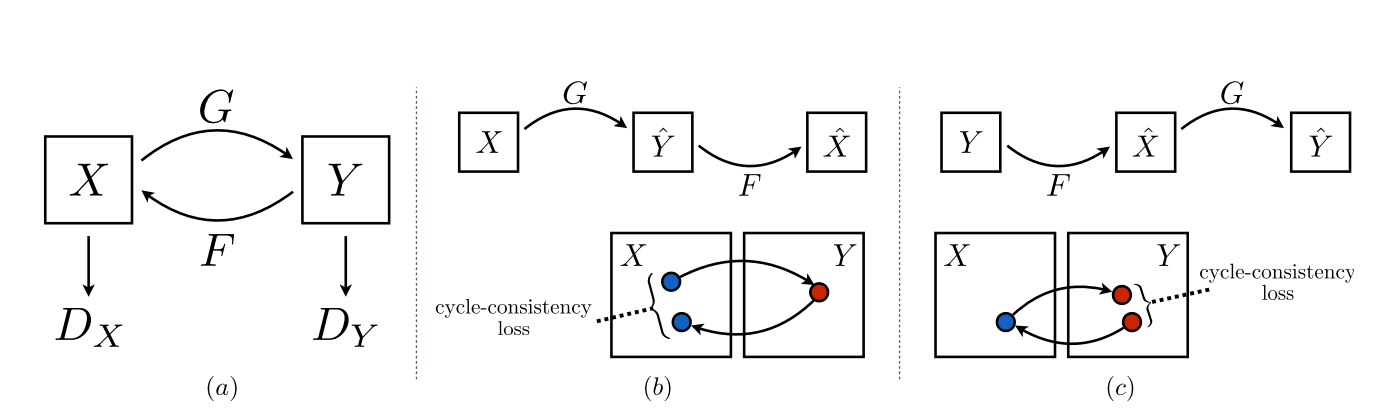As illustrated in the figure, the model includes two mappings G: X → Y and F: Y → X. Besides, two adversarial discriminators are introduced, DX and DY; task of DX is to discriminate images x from translated images F(y), whereas DY aims to discriminate y from G(x). So, the final objective has two different loss terms: adversarial loss for matching the distribution of generated images to the data distribution in the target domain, and cycle consistency loss to prevent the learned mappings G and F from contradicting each other.

### Loss Formulation

Adversarial loss is applied to both the mapping functions - G: X → Y and F: Y → X. G tries to generate images G(x) that look similar to images from domain Y, and DY tries to distinguish the translated samples G(x) from real samples y (similar argument holds for the other one).

• Generator (G) tries to minimize: ` E[x∼pdata(x)] (D(G(x)) − 1)2 `
• Discriminator (DY) tries to minimize: ` E[y∼pdata(y)] (D(y) − 1)2 + E[x∼pdata(x)] D(G(x))2 `
• Generator (F) tries to minimize ` E[y∼pdata(y)] (D(G(y)) − 1)2 `
• Discriminator (DX) tries to minimize: ` E[x∼pdata(x)] (D(x) − 1)2 + E[y∼pdata(y)] D(G(y))2 `

#### Cycle Consistency Loss:

Adversarial training can, in theory, learn mappings G and F that produce outputs identically distributed as target domains Y and X respectively (strictly speaking, this requires G and F to be stochastic functions). However, with large enough capacity, a network can map the same set of input images to any random permutation of images in the target domain, where any of the learned mappings can induce an output distribution that matches the target distribution. Thus, adversarial losses alone cannot guarantee that the learned function can map an individual input xi to a desired output yi. To further reduce the space of possible mapping functions, learned functions should be cycle-consistent. ` Lcyc (G, F) = E[x∼pdata(x)] || F(G(x)) − x|| + E[y∼pdata(y)] || G(F(y)) − y || `

#### Full Objective:

The full objective is: ` L (G, F, DX, DY) = LGAN (G, DY , X, Y) + LGAN (F, DX, Y, X) + λ Lcyc (G, F) ` , where lambda controls the relative importance of the two objectives. λ is set to 10 in the final loss equation. For painting → photo, authors found that it was helpful to introduce an additional loss to encourage the mapping to preserve color composition between the input and output. In particular, they regularized the generator to be near an identity mapping when real samples of the target domain are provided as the input to the generator i.e., ` Lidentity (G, F) = E[y∼pdata(y)] || G(y) − y || + E[x∼pdata(x)] || F(x) − x ||. `

#### Key Takeaways:

• It is difficult to optimize adversarial objective in isolation - standard procedures often lead to the well-known problem of mode collapse. Both the mappings G and F are trained simultaneously to enforce the structural assumption.

• The translation should be Cycle consistent; mathematically, translator G: X → Y and another translator F: Y → X, should be inverses of each other (and both mappings should be bijections).

• It is similar to training two autoencoders - F ◦ G: X → X jointly with G ◦ F: Y → Y. These autoencoders have special internal structure - map an image to itself via an intermediate repr that is a translation of the image into another domain.

• It can also be treated as a special case of adversarial autoencoders , which use an adversarial loss to train the bottleneck layer of an autoencoder to match an arbitrary target distribution.

### Network Architecture

#### Generator:

Authors adopted the Generator's architecture from the neural style transfer and super-resolution paper. The network contains two stride-2 convolutions, several residual blocks, and two fractionally-strided convolutions with stride 1/2. 6 or 9 ResBlocks are used in the generator depending on the size of the training images. Instance normalization is used instead of batch normalization. ` 128 x 128 images: c7s1-64, d128, d256, R256, R256, R256, R256, R256, R256, u128, u64, c7s1-3 ` ` 256 x 256 images: c7s1-64, d128, d256, R256, R256, R256, R256, R256, R256, R256, R256, R256, u128, u64, c7s1-3 `

#### Discriminator:

The same 70 x 70 PatchGAN discriminator is used, which aims to classify whether 70 x 70 overlapping image patches are real or fake (more parameter efficient compared to full-image discriminator). To reduce model oscillations, discriminators are updated using a history of generated images rather than the latest ones with a probability of 0.5.` 70 x 70 PatchGAN: C64-C128-C256-C512 `

>

c7s1-k denote a 7×7 Convolution - InstanceNorm - ReLU Layer with k filters and stride 1. dk denotes a 3 × 3 Convolution - InstanceNorm - ReLU layer with k filters and stride 2. Reflection padding is used to reduce artifacts. Rk denotes a residual block that contains two 3 × 3 convolutional layers with the same number of filters on both layer. uk denotes a 3 × 3 Deconv - InstanceNorm - ReLU layer with k filters and stride 1/2. Ck denote a 4 × 4 Convolution - InstanceNorm - LeakyReLU layer with k filters and stride 2. After the last layer, a convolution is applied to produce a 3-channels output for generator and 1-channel output for discriminator. No InstanceNorm in the first C64 layer.

### Results:

#### Photo -> Cezzane Paintings: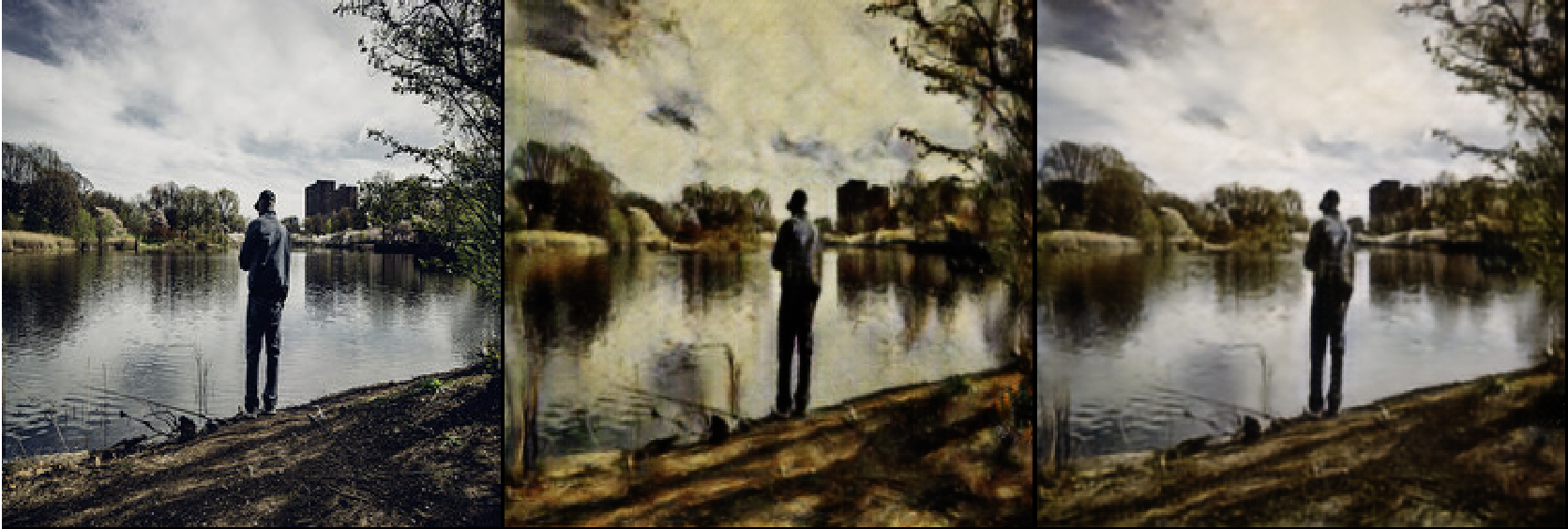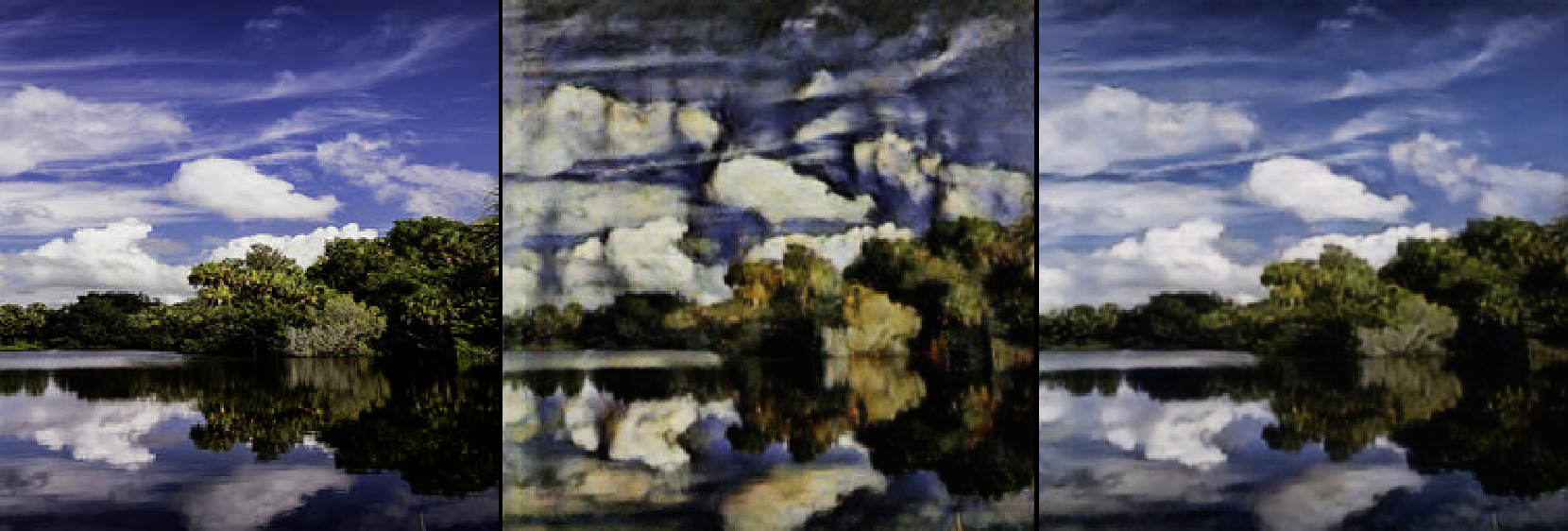#### Cezzane Paintings -> Photo: